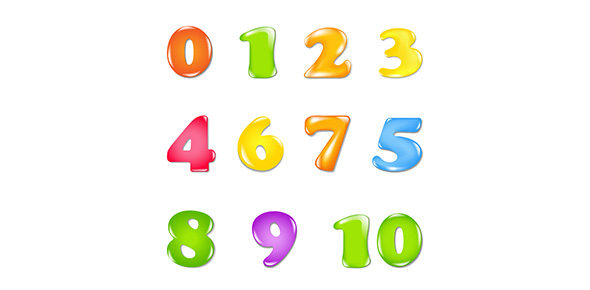# Section 1.5 - Rational Number Operations

9 Questions | Attempts: 249SettingsComplete the following questions

• 1.
Calculate the expression: -3 2/3 + (-6 2/5)
• A.

-10 1/15

• B.

-9 14/15

• C.

-2 11/15

• D.

10 1/15

• 2.
When you multiply two numbers, which of the following will result in a negative product?
• A.

If both factors are negative

• B.

If one factor is negative and the other is 0

• C.

If both factors are positive

• D.

If one factor is negative and the other is postive

• 3.
The temperature outside is -10 F.  What is the temperature is degrees Celsius?  Use the formula C = 5/9(F - 32) to convert to degrees Celsius.
• A.

-75.2 C

• B.

-23 1/3 C

• C.

-12 2/9 C

• D.

12 2/9 C

• 4.
Evaluate the expression (3/5 - 5/8) x (-2/3)
• A.

-1/30

• B.

-1/60

• C.

1/60

• D.

1/30

• 5.
Evaluate the expression: -4.5(2.8)(3.6 - 1.9)
• A.

-34.02

• B.

-21.42

• C.

21.42

• D.

34.02

• 6.
At a golf tournament the golfers has a mean score of -2.25.  If there were 60 golfers in the tournament, what was their combined score?
• A.

-150

• B.

-135

• C.

135

• D.

150

• 7.
Evaluate the expression:  2 3/8 x (-5/6) / (3/4)
• A.

-3 7/36

• B.

-2 23/36

• C.

-1 31/64

• D.

-5/19

• 8.
What number makes this equation true:  -2 1/2 x ____ = - 1
• A.

-1 1/2

• B.

-2/5

• C.

2/5

• D.

1 1/2

• 9.
The low temperature was - 25 C in January.  What was the low temperature in degrees Fahrenheit?  Use the formula F = 9/5C + 32 to convert to degrees Fahrenheit
• A.

-102.6 F

• B.

-13 F

• C.

8.8 F

• D.

12.6 F

## Related TopicsBack to top
×

Wait!
Here's an interesting quiz for you.